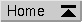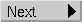# The Circles PuzzleThis is a geometry puzzle wich appeared in May 1996 in the newsgroup rec.puzzles. The original statement was:

"What is the smallest circle running through twelve lattice points?"

The obvious answer (a circle with radius 5, centered at a lattice node, e.g. the origin) was found quite soon, but this isn't the smallest such circle.

Soon thereafter, someone extended the problem statement to

"Are there any circles running through an odd number of lattice points? And what is the smallest circle running through 20 lattice points?"

For the first question, I found a solution by hand: it's a circle centered e.g. at `(7/6, 5/6)` with a radius of `5 * sqrt (2) / 6`, running through points `(0, 1)`, `(1, 2)`, and `(2, 0)`. (Note that this is a smaller circle than the one that's even easier to find: centered at `(0, 1/4)` with a radius of `5/4` and going through lattice points `(0, -1)`, `(-1, 1)`, and `(1, 1)`.) The solutions for circles running through exactly 2, 4, 6, 8, 12, or 16 points also were quite easy to find.

But then I got interested in finding out whether there were any circles running through other odd numbers of lattice points. How about 5, 7, or 9?

I hacked together a small program which simply takes all possible combinations of the origin plus two other points (not collinear, of course) within a square centered at the origin, calculated the center of the circle defined by these three points and then did a brute force check to see how many other points there were on the perimeter of the circle.

The results were quite surprising!